# LINQ Union Method in C#

## LINQ Union Method in C# with Examples

In this article, I will discuss the LINQ Union Method in C# with Examples. Please read our previous article discussing the LINQ Intersect Method in C# with Examples. As part of this article, we will discuss the following pointers related to the LINQ Union method in C#.

1. What is the LINQ Union Method?
2. Examples to Understand LINQ Union Method with Value Type in C#
3. What happens if any sequences are null while performing the Union Operation?
4. LINQ Union Method Example with String Array using C#
5. LINQ Union Method with Complex Type in C#
6. Using IEqualityComparer Comparer with LINQ Union Method in C#
7. Using Anonymous Type with Union Method in C#
8. Overriding Equals() and GetHashCode() Methods of Object Class
9. Implementing IEquatble<T> Interface in C#
10. When to Use the LINQ Union Method in Real-time Applications?
##### What is the LINQ Union Method in C#?

The LINQ Union Method in C# combines two sequences (collections) into one sequence while removing duplicate elements. It’s similar to the UNION operation in SQL and is used to produce a set of unique elements from the two collections.

The Union Method in LINQ is an extension method for types that implement IEnumerable<T>, where T is the type of elements in the sequences. This method resides in the System.Linq namespace. As shown in the image below, two overloaded versions are available for the LINQ Union Method.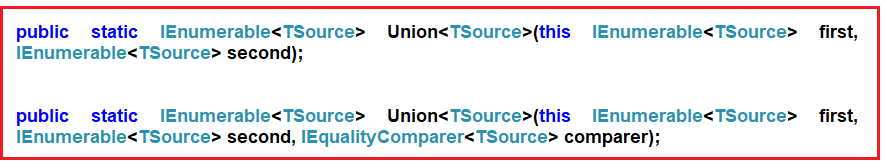The one and only difference between the above two LINQ Union methods is that the second overloaded version takes IEqualityComparer as an argument. That means when working with Complex Data Types, we can use the overloaded method to work as expected, which takes the IEqualityComparer parameter.

##### Examples to Understand LINQ Union Method with Value Type in C#

Let us understand the LINQ Union Method in C# with an Example. Please have a look at the below image. As you can see in the image below, we have two integer data sources, i.e., DataSource 1 and DataSource 2. DataSource 1 contains elements 1, 2, 3, 4, 5, and 6, and DataSource 2 contains elements 1, 3, 5, 8, 9, and 10. If we want to retrieve all the elements from both collections by removing the duplicate element, i.e., if we want the result as 1, 2, 3, 4, 5, 6, 8, 9, and 10, then we need to use the LINQ Union Method.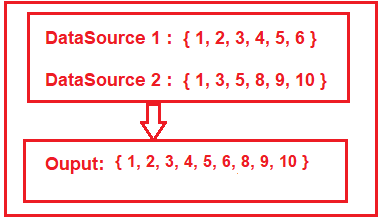##### LINQ Union Method Example using Method and Query Syntax:

The following example shows the LINQ Union Method using both Method and Query Syntax to fetch all elements from both collections by removing the duplicate elements. In LINQ Query Syntax, no such operator called Union is available, so here, we need to use the mixed syntax, i.e., both the query and method syntax, to achieve the same.

```using System.Collections.Generic;
using System;
using System.Linq;
namespace LINQDemo
{
class Program
{
static void Main(string[] args)
{
List<int> dataSource1 = new List<int>() { 1, 2, 3, 4, 5, 6 };
List<int> dataSource2 = new List<int>() { 1, 3, 5, 8, 9, 10 };

//Method Syntax
var MS = dataSource1.Union(dataSource2).ToList();

//Query Syntax
var QS = (from num in dataSource1
select num)
.Union(dataSource2).ToList();

foreach (var item in MS)
{
Console.WriteLine(item);
}

}
}
}```

Run the application, and you will see the output as expected, i.e., 1 2 3 4 5 6 8 9 10

##### What happens if any sequences are null while performing the Union Operation?

The Union Method will throw an exception if any sequence is null. In the below example, the second sequence is null, and while performing the Union operation using the Union Method, it will throw an exception.

```using System.Collections.Generic;
using System;
using System.Linq;
namespace LINQDemo
{
class Program
{
static void Main(string[] args)
{
List<int> dataSource1 = new List<int>() { 1, 2, 3, 4, 5, 6 };
List<int> dataSource2 = null;

//Method Syntax
var MS = dataSource1.Union(dataSource2).ToList();

foreach (var item in MS)
{
Console.WriteLine(item);
}

}
}
}```

Now run the application, and you will get the following exception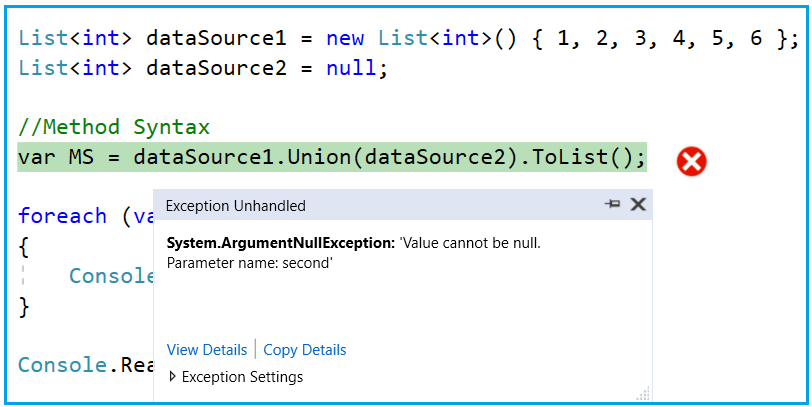##### LINQ Union Method Example with String Array using C#:

Let us see an example to understand how the LINQ Union Method works with string collection in C#. Here, in the below example, we have two arrays of countries that also contain the same country name, and our requirement is to return all countries from both collections by removing duplicate country names.

```using System;
using System.Linq;
namespace LINQDemo
{
class Program
{
static void Main(string[] args)
{
string[] dataSource1 = { "India", "USA", "UK", "Canada", "Srilanka" };
string[] dataSource2 = { "India", "uk", "Canada", "France", "Japan" };

//Method Syntax
var MS = dataSource1.Union(dataSource2).ToList();

//Query Syntax
var QS = (from country in dataSource1
select country)
.Union(dataSource2).ToList();

foreach (var item in MS)
{
Console.WriteLine(item);
}

}
}
}```

Now, run the application, and it will give us the following output.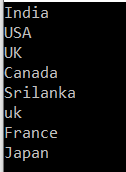As you can see, it displays the country UK twice. This is because the default comparer that is being used by the LINQ Union method is case-insensitive. So, if you want to ignore the case-sensitive, you need to use the other overloaded version of the Union method, which takes IEqualityComparer as an argument. Let us modify the Main method of the program class as follows to use the StringComparer as an argument that implements the IEqualityComparer interface.

```using System;
using System.Linq;
namespace LINQDemo
{
class Program
{
static void Main(string[] args)
{
string[] dataSource1 = { "India", "USA", "UK", "Canada", "Srilanka" };
string[] dataSource2 = { "India", "uk", "Canada", "France", "Japan" };

//Method Syntax
var MS = dataSource1.Union(dataSource2, StringComparer.OrdinalIgnoreCase).ToList();

//Query Syntax
var QS = (from country in dataSource1
select country)
.Union(dataSource2, StringComparer.OrdinalIgnoreCase).ToList();

foreach (var item in MS)
{
Console.WriteLine(item);
}

}
}
}
```

Now run the application, and it should display the data as expected, as shown in the below image.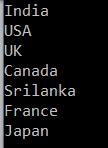##### LINQ Union Method with Complex Type in C#:

The LINQ Union() Method, like other Set Methods (such as Distinct, Expect, and Intersect), also works differently when working with complex data types such as Product, Employee, Student, etc. Let us understand this with an example. Create a class file named Student.cs and copy and paste the following code.

```namespace LINQDemo
{
public class Student
{
public int ID { get; set; }
public string Name { get; set; }
}
}
```

The Student class has two properties, i.e., ID and Name. Let’s say we have the following two data sources containing duplicate data.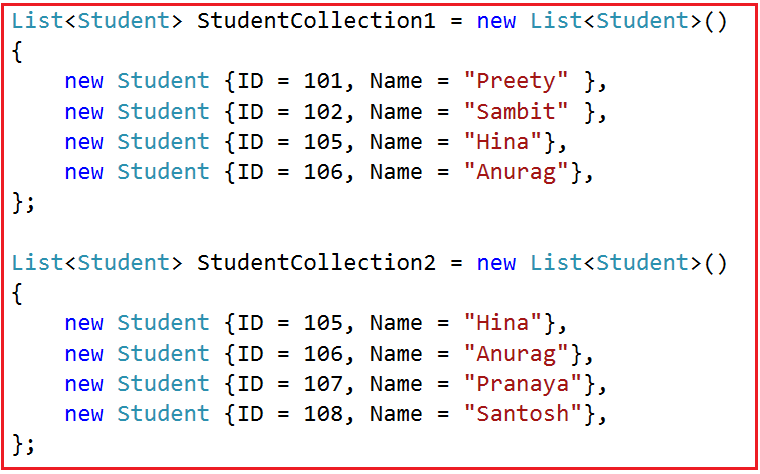As you can see in the above image, we have two student data collections. And if you notice, we have two students, Hina and Anurag, who appeared in both collections. Our requirement is to fetch all the student names from both collections by removing the duplicate names. That means we want the names Hina and Anurag to appear only once in the result. To achieve the same, we need to use the LINQ Union Method.

So, modify the Main method of the Program class as follows. In the example below, from the first data source, we are fetching the student names by using the select method, then we use the Union method, and then we are fetching the student names from the second data source.

```using System.Collections.Generic;
using System;
using System.Linq;
namespace LINQDemo
{
class Program
{
static void Main(string[] args)
{
List<Student> StudentCollection1 = new List<Student>()
{
new Student {ID = 101, Name = "Preety" },
new Student {ID = 102, Name = "Sambit" },
new Student {ID = 105, Name = "Hina"},
new Student {ID = 106, Name = "Anurag"},
};

List<Student> StudentCollection2 = new List<Student>()
{
new Student {ID = 105, Name = "Hina"},
new Student {ID = 106, Name = "Anurag"},
new Student {ID = 107, Name = "Pranaya"},
new Student {ID = 108, Name = "Santosh"},
};

//Method Syntax
var MS = StudentCollection1.Select(x => x.Name)
.Union(StudentCollection2.Select(y => y.Name)).ToList();

//Query Syntax
var QS = (from std in StudentCollection1
select std.Name)
.Union(StudentCollection2.Select(y => y.Name)).ToList();

foreach (var name in MS)
{
Console.WriteLine(name);
}

}
}
}```
###### Output:

When you run the above code, you will get the output as expected, as shown in the below image. Here, you can see the names Hina and Anurag appeared only once.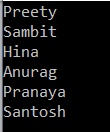Our requirement has changed, and we need to select all the students’ information from both collections by removing the duplicate students. To do this, let us modify the Main Method of the Program class as shown below.

```using System.Collections.Generic;
using System;
using System.Linq;
namespace LINQDemo
{
class Program
{
static void Main(string[] args)
{
List<Student> StudentCollection1 = new List<Student>()
{
new Student {ID = 101, Name = "Preety" },
new Student {ID = 102, Name = "Sambit" },
new Student {ID = 105, Name = "Hina"},
new Student {ID = 106, Name = "Anurag"},
};

List<Student> StudentCollection2 = new List<Student>()
{
new Student {ID = 105, Name = "Hina"},
new Student {ID = 106, Name = "Anurag"},
new Student {ID = 107, Name = "Pranaya"},
new Student {ID = 108, Name = "Santosh"},
};

//Method Syntax
var MS = StudentCollection1.Union(StudentCollection2).ToList();

//Query Syntax
var QS = (from std in StudentCollection1
select std).Union(StudentCollection2).ToList();

foreach (var student in MS)
{
Console.WriteLine(\$" ID : {student.ID} Name : {student.Name}");
}

}
}
}```

Once you run the application, you will see that it displays all the students without removing the duplicate students, as shown in the below image.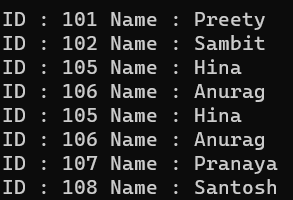As you can see in the above output, the students Hina and Anurag appeared twice. This is because the default compare, used for comparison by the LINQ Union Method, only checked whether two object references are equal and not the individual property values of the complex object. As discussed in our previous three articles, we can overcome this problem in many ways. Let us understand each way one by one.

##### Using IEqualityComparer Comparer with LINQ Union Method in C#:

In this approach, we need to create a class and then implement the IEqualityComparer interface and provide the implementation for the Equals and GetHashCode methods. So, create a class file named StudentComparer.cs and copy and paste the following code.

```using System.Collections.Generic;
namespace LINQDemo
{
public class StudentComparer : IEqualityComparer<Student>
{
public bool Equals(Student x, Student y)
{
return x.ID == y.ID && x.Name == y.Name;
}

public int GetHashCode(Student obj)
{
return obj.ID.GetHashCode() ^ obj.Name.GetHashCode();
}
}
}
```

Next, we need to create an instance of the StudentComparer class, and then we need to pass that instance to the Union method, which takes IEqualityComparer as a parameter. So, modify the Main method of the Program class as follows.

```using System.Collections.Generic;
using System;
using System.Linq;
namespace LINQDemo
{
class Program
{
static void Main(string[] args)
{
List<Student> StudentCollection1 = new List<Student>()
{
new Student {ID = 101, Name = "Preety" },
new Student {ID = 102, Name = "Sambit" },
new Student {ID = 105, Name = "Hina"},
new Student {ID = 106, Name = "Anurag"},
};

List<Student> StudentCollection2 = new List<Student>()
{
new Student {ID = 105, Name = "Hina"},
new Student {ID = 106, Name = "Anurag"},
new Student {ID = 107, Name = "Pranaya"},
new Student {ID = 108, Name = "Santosh"},
};

StudentComparer studentComparer = new StudentComparer();

//Method Syntax
var MS = StudentCollection1
.Union(StudentCollection2, studentComparer).ToList();

//Query Syntax
var QS = (from std in StudentCollection1
select std)
.Union(StudentCollection2, studentComparer).ToList();

foreach (var student in MS)
{
Console.WriteLine(\$" ID : {student.ID} Name : {student.Name}");
}

}
}
}
```

With the above changes in place, run the application, and you will get the output as expected, as shown in the below image. Here, you can see Hina and Anurag appeared only once.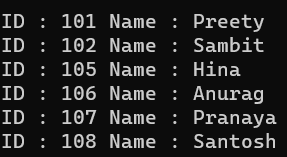##### Using Anonymous Type with Union Method in C#:

In this approach, we must select all the individual properties to an anonymous type using the LINQ select operator or Select Extension Method. The following program does exactly the same thing.

```using System.Collections.Generic;
using System;
using System.Linq;
namespace LINQDemo
{
class Program
{
static void Main(string[] args)
{
List<Student> StudentCollection1 = new List<Student>()
{
new Student {ID = 101, Name = "Preety" },
new Student {ID = 102, Name = "Sambit" },
new Student {ID = 105, Name = "Hina"},
new Student {ID = 106, Name = "Anurag"},
};

List<Student> StudentCollection2 = new List<Student>()
{
new Student {ID = 105, Name = "Hina"},
new Student {ID = 106, Name = "Anurag"},
new Student {ID = 107, Name = "Pranaya"},
new Student {ID = 108, Name = "Santosh"},
};

//Method Syntax
var MS = StudentCollection1.Select(x => new { x.ID, x.Name })
.Union(StudentCollection2.Select(x => new { x.ID, x.Name })).ToList();

//Query Syntax
var QS = (from std in StudentCollection1
select new { std.ID, std.Name })
.Union(StudentCollection2.Select(x => new { x.ID, x.Name })).ToList();

foreach (var student in MS)
{
Console.WriteLine(\$" ID : {student.ID} Name : {student.Name}");
}

}
}
}
```

With the above changes in place, now run the application, and you will get the output as expected, as shown in the below image.##### Overriding Equals() and GetHashCode() Methods of Object Class

This is the third approach, and in this approach, we need to override the Equals and GetHashCode() in the Student class. By default, any type (predefined or user-defined) .NET Framework is inherited from the Object class. That means the Student class is also inherited from the Object class. So, we can override the Equals() and GetHashCode() methods of the Object class within the Student class. So, modify the Student class as shown below. We are overriding the Equals() and GetHashCode() methods here.

```namespace LINQDemo
{
public class Student
{
public int ID { get; set; }
public string Name { get; set; }

public override bool Equals(object obj)
{
//As the obj parameter type is object, so we need to
//cast it to Student Type
return this.ID == ((Student)obj).ID && this.Name == ((Student)obj).Name;
}

public override int GetHashCode()
{
//Get the ID hash code value
int IDHashCode = this.ID.GetHashCode();
//Get the string HashCode Value
//Check for null refernece exception
int NameHashCode = this.Name == null ? 0 : this.Name.GetHashCode();
return IDHashCode ^ NameHashCode;
}
}
}
```

Next, we need to modify the Main method of the Program class, as shown below. Here, we need to use the overloaded version of the Union method, which does not take any parameter.

```using System.Collections.Generic;
using System;
using System.Linq;
namespace LINQDemo
{
class Program
{
static void Main(string[] args)
{
List<Student> StudentCollection1 = new List<Student>()
{
new Student {ID = 101, Name = "Preety" },
new Student {ID = 102, Name = "Sambit" },
new Student {ID = 105, Name = "Hina"},
new Student {ID = 106, Name = "Anurag"},
};

List<Student> StudentCollection2 = new List<Student>()
{
new Student {ID = 105, Name = "Hina"},
new Student {ID = 106, Name = "Anurag"},
new Student {ID = 107, Name = "Pranaya"},
new Student {ID = 108, Name = "Santosh"},
};

//Method Syntax
var MS = StudentCollection1.Union(StudentCollection2).ToList();

//Query Syntax
var QS = (from std in StudentCollection1
select std).Union(StudentCollection2).ToList();

foreach (var student in MS)
{
Console.WriteLine(\$" ID : {student.ID} Name : {student.Name}");
}

}
}
}
```

Now run the application code, and you will get the same output as the previous two examples as expected, as shown in the below image.##### Implementing IEquatble<T> Interface in C#

In this approach, we need to implement the IEquatble<T> Interface in Student Class, and we need to implement the Equals Method of the IEquatble<T> Interface. We also need to override the GetHashCode method of the Object class. So, modify the Student class as shown below.

```using System;
namespace LINQDemo
{
public class Student : IEquatable<Student>
{
public int ID { get; set; }
public string Name { get; set; }

public bool Equals(Student other)
{
return this.ID.Equals(other.ID) && this.Name.Equals(other.Name);
}
public override int GetHashCode()
{
int IDHashCode = this.ID.GetHashCode();
int NameHashCode = this.Name == null ? 0 : this.Name.GetHashCode();
return IDHashCode ^ NameHashCode;
}
}
}
```

Here, first, we implement the Equals method of the IEquatable interface, and then we override the GetHashCode method of the Object class. With the above changes in place, now modify the Main Method of the Program class as shown below.

```using System.Collections.Generic;
using System;
using System.Linq;
namespace LINQDemo
{
class Program
{
static void Main(string[] args)
{
List<Student> StudentCollection1 = new List<Student>()
{
new Student {ID = 101, Name = "Preety" },
new Student {ID = 102, Name = "Sambit" },
new Student {ID = 105, Name = "Hina"},
new Student {ID = 106, Name = "Anurag"},
};

List<Student> StudentCollection2 = new List<Student>()
{
new Student {ID = 105, Name = "Hina"},
new Student {ID = 106, Name = "Anurag"},
new Student {ID = 107, Name = "Pranaya"},
new Student {ID = 108, Name = "Santosh"},
};

//Method Syntax
var MS = StudentCollection1.Union(StudentCollection2).ToList();

//Query Syntax
var QS = (from std in StudentCollection1
select std).Union(StudentCollection2).ToList();

foreach (var student in MS)
{
Console.WriteLine(\$" ID : {student.ID} Name : {student.Name}");
}

}
}
}
```

Now, run the application, and you will also get the same output as expected, as shown in the below image.##### When to use the LINQ Union Method in C#?

The LINQ Union method in C# is useful when combining two or more collections while ensuring the resulting collection contains unique elements. Here are some common scenarios when you might want to use the Union method:

• Combining Collections Without Duplicates: When you have two collections and you want to create a single collection that contains a unique set of elements from both, Union is the appropriate choice.
• Data Consolidation: Useful in data processing, data from multiple sources or collections must be consolidated into a single collection without repeating any element.
• Creating Distinct Sets: In scenarios where you deal with sets and need to perform set operations. Union is the equivalent of the mathematical union of sets.
• Merging Data with Overlaps: When merging data that might have overlapping elements, you need to ensure each element only appears once in the merged collection.

In the next article, I will discuss the LINQ Concat Method using C# with Examples. In this article, I explain the LINQ Union Method using C# with Examples. I hope you enjoy this article and understand the concept of the LINQ Union Method in C# with Examples.